Next: 6.2 Muonium Diffusion in Up: 6 Quantum Diffusion II: Previous: 6 Quantum Diffusion II:

# 6.1 Two-Phonon Quantum Diffusion

At temperatures well below the Debye temperature of the host solid, the tunnelling diffusion rate will be determined by the full coherent transition amplitude as long as the rate of loss of phase coherence,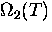, is small compared to the inverse of the underbarrier transition time. Without scattering, the mean free path can exceed the lattice constant so the particle propagates in a band-like state. As the temperature is increased, two things happen: the enhanced phonon population causes a very rapid increase in,suppressing the coherent channel; meanwhile the hop rate due to the one-phonon, thermally-activated tunnelling mechanism increases exponentially. The contribution of one-phonon hopping becomes dominant when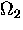exceeds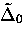, providing a natural division of the temperature scale. For the purpose of discussing quantum diffusion, low'' temperature means temperatures where the 2-phonon incoherent channel, though partly diminished by phonon scattering, still exceeds the contribution of thermally-activated hopping mediated by the one-phonon interaction. In this section we will consider in more detail the predictions of theory in the incoherent regime and compare these with experimental results.

Previously we showed that the incoherent diffusion rate in the presence of an interstitial-lattice coupling quadratic in lattice displacements (i.e. two-phonon coupling) is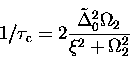(1)
where the tunnelling bandwidth renormalized by the one-phonon interaction is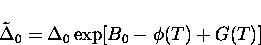(2)
with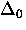the bandwidth in a perfect lattice and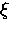the typical site-to-site static level shift. The temperature dependent parameters in the exponent characterize the polaron effect and barrier fluctuations and are respectively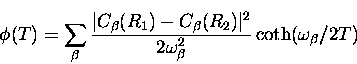(3)
and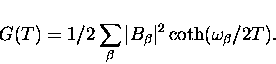(4)
The de-coherence ratewas defined in terms of the two-phonon coupling parameters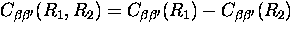and phonon numbers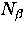by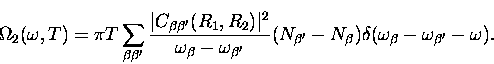(5)

Recalling that the largest contribution from phonon scattering comes from those terms where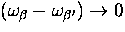, we can write the difference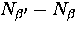in this limit as

In this limit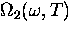becomes frequency-independent and is given by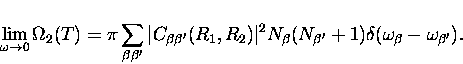(6)
Frequency independence of the damping rateimplies that two-phonon scattering is an example of an Ohmic coupling to the medium in the Caldeira-Leggett theory of dissipation. [38,39] In order to obtain the hop rate as an explicit function of temperature we approximate the summation over phonons by an integral over the phonon density of states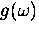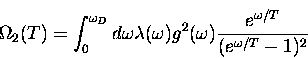(7)
where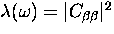is the matrix element in Eq. (6.5) averaged over the branches of the phonon modes.

At this stage it is possible to determine the temperature dependence of the hop rate at least qualitatively for temperatures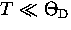.Solids generally have phonon spectra that at long wavelengths - the only modes excited at low T - closely follow the Debye model with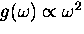and the coupling constant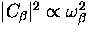.In the low temperature limit Eq. (6.8) then gives us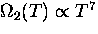.

We are now in a position to consider the qualitative temperature dependence of the interstitial diffusion rate. In the case that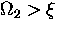, Eq. (6.1) reduces approximately to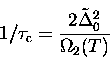(8)
and where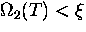to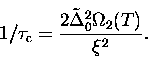(9)
Sincealways increases monotonically with temperature we have two possible situations. We expect that at sufficiently low temperatures (but still large compared to the bandwidth) there is the possibility that Eq. (6.10) applies and in the low temperature limitwe have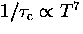. In this case the scattering of phonons actually helps the diffusing particle to overcome the static level shifts.The other possibility (generally at some higher temperature) is that the static shifts are comparatively small in which case Eq. (6.9) applies and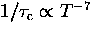; phonon scattering then hinders the band-like diffusion in the periodic potential of the lattice.Next: 6.2 Muonium Diffusion in Up: 6 Quantum Diffusion II: Previous: 6 Quantum Diffusion II: